## 11.2.3 Probabilistic Information Spaces

This section defines the I-map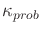from Figure 11.3, which converts each history I-state into a probability distribution over. A Markov, probabilistic model is assumed in the sense that the actions of nature only depend on the current state and action, as opposed to state or action histories. The set union and intersection of (11.30) and (11.31) are replaced in this section by marginalization and Bayes' rule, respectively. In a sense, these are the probabilistic equivalents of union and intersection. It will be very helpful to compare the expressions from this section to those of Section 11.2.2.

Rather than write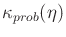, standard probability notation will be applied to obtain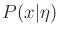. Most expressions in this section of the form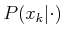have an analogous expression in Section 11.2.2 of the form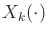. It is helpful to recognize the similarities.

The first step is to construct probabilistic versions of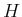and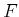. These are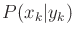and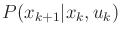, respectively. The latter term was given in Section 10.1.1. To obtain, recall from Section 11.1.1 that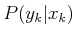is easily derived from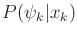. To obtain, Bayes' rule is applied: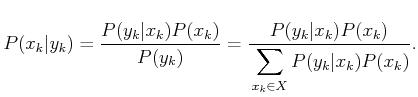(11.35)

In the last step,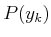was rewritten using marginalization, (9.8). In this case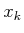appears as the sum index; therefore, the denominator is only a function of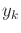, as required. Bayes' rule requires knowing the prior,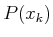. In the coming expressions, this will be replaced by a probabilistic I-state.

Now consider defining probabilistic I-states. Each is a probability distribution overand is written as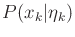. The initial condition produces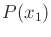. As for the nondeterministic case, probabilistic I-states can be computed inductively. For the base case, the only new piece of information is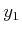. Thus, the probabilistic I-state,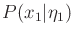, is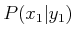. This is computed by letting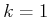in (11.35) to yield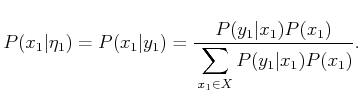(11.36)

Now consider the inductive step by assuming thatis given. The task is to determine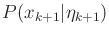, which is equivalent to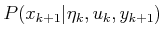. As in Section 11.2.2, this will proceed in two parts by first considering the effect of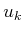, followed by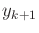. The first step is to determine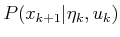from. First, note that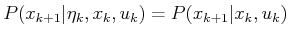(11.37)

because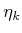contains no additional information regarding the prediction of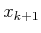onceis given. Marginalization, (9.8), can be used to eliminatefrom. This must be eliminated because it is not given. Putting these steps together yields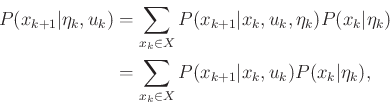(11.38)

which expressesin terms of given quantities. Equation (11.38) can be considered as the probabilistic counterpart of (11.30).

The next step is to take into account the observation. This is accomplished by making a version of (11.35) that is conditioned on the information accumulated so far:and. Also,is replaced with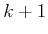. The result is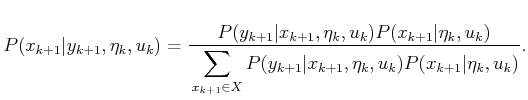(11.39)

This can be considered as the probabilistic counterpart of (11.31). The left side of (11.39) is equivalent to, which is the probabilistic I-state for stage, as desired. There are two different kinds of terms on the right. The expression foris given in (11.38). Therefore, the only remaining term to calculate is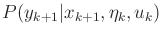. Note that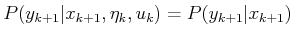(11.40)

because the sensor mapping depends only on the state (and the probability model for the nature sensing action, which also depends only on the state). Since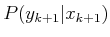is specified as part of the sensor model, we have now determined how to obtainfrom,, and. Thus,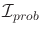is another I-space that can be treated as just another state space.

The probabilistic I-space(shown in Figure 11.3) is the set of all probability distributions over. The update expressions, (11.38) and (11.39), establish that the I-mapis sufficient, which means that the planning problem can be expressed entirely in terms of, instead of maintaining histories. A goal region can be specified as constraints on the probabilities. For example, from some particular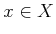, the goal might be to reach any probabilistic I-state for which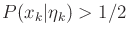.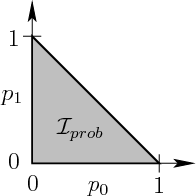Example 11..14 (Three-State Example Revisited)   Now return to Example 11.13, but this time use probabilistic models. For a probabilistic I-state, let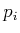denote the probability that the current state is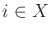. Any probabilistic I-state can be expressed as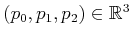. This implies that the I-space can be nicely embedded in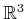. By the axioms of probability (given in Section 9.1.2),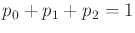, which can be interpreted as a plane equation inthat restrictsto a 2D set. Also following the axioms of probability, for each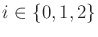,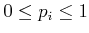. This means thatis restricted to a triangular region in. The vertices of this triangular region are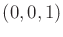,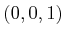, and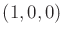; these correspond to the three different ways to have perfect state information. In a sense, the distance away from these points corresponds to the amount of uncertainty in the state. The uniform probability distribution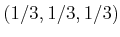is equidistant from the three vertices. A projection of the triangular region into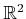is shown in Figure 11.6. The interpretation in this case is that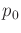and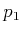specify a point in, and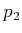is automatically determined from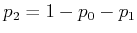.

The triangular region inis an uncountably infinite set, even though the history I-space is countably infinite for a fixed initial condition. This may seem strange, but there is no mistake because for a fixed initial condition, it is generally impossible to reach all of the points in. If the initial condition can be any point in, then all of the probabilistic I-space is covered because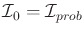, in which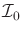is the initial condition space..Steven M LaValle 2020-08-14